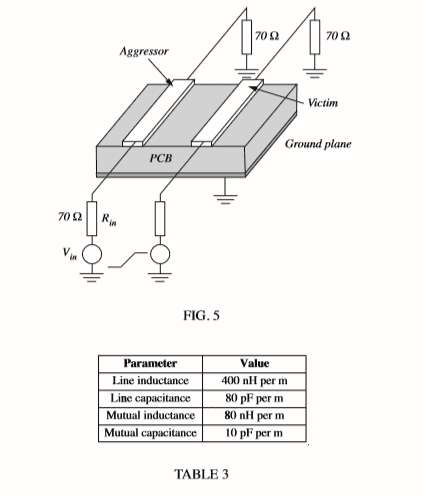# Help with NEXT and FEXT volatage magnitudes

## Homework Statement

A transmission line is formed by two identical parallel tracks in a printed circuit board. The line has a length of 50 mm and all line terminations are of 70 Ω. The line can be assumed to be lossless.

calculate the magnitude of the NEXT and FEXT voltages generated in the victim conductor when the source voltage, Vin, in the aggressor conductor is a voltage step of 2 V with a rise time of 100 ps.

## Homework Equations

Vs = Vin x Rin/(Rin+Zo)
Kne =Vp/4 (CmZo + Lm/Zo)
Vne = Kne (Vs(t) - Vs(t-2td)
Kfe = 1/2(CmZo - Lm/Zo)
Vfe = Kfe x length (d/dt)[Vs(t-td)]

## The Attempt at a Solution

Zo = root L/C = 70.7Ohms
Vp = 1/root LC = 17677695
Vs = Vin x Rin/(Rin+Zo) = 0.995v
Kne =Vp/4 (CmZo + Lm/Zo) = 0.08

I start to struggle with the next part (assuming the previous are correct) in regard to t and 2td. I'm sure from my notes t=vp but I'm unable to find the page any more and so I'm unable to verify this.

Vne = Kne (Vs(t) - Vs(t-2td) = 0.08

Again I'm struggling to find d and dt in regard to Far end

Kfe = 1/2(CmZo - Lm/Zo) = 3.99515E9

Vfe = Kfe x length (d/dt)[Vs(t-td)]

Any help would be much appreciated.

Baluncore
2021 Award
How many transmission lines do you have?
One line made from two parallel tracks, fed with a differential signal, or;
Two lines over a ground plane that work as a backward wave coupler?
All voltages are differential. What is/are the reference voltage(s).
FEXT = Far end cross talk, from what to what, relative to what?
NEXT = Near end cross talk, from what to what, relative to what?

•berkemanThe above picture accompanied the original question this is all the information ive been given.

#### Attachments

You sir are a gentleman and a scholar, thank you very much!

is t the rise time? 100ps?

I am thinking not, because later in the notes it expresses td to be half the rise time.

If so what is t? for both the NEXT and FEXT equations??

thanks
Craig

is it this:

t = length √LV
td = Tr / 2